International
Tables for
Crystallography
Volume B
Reciprocal space
Edited by U. Shmueli

International Tables for Crystallography (2006). Vol. B. ch. 4.5, pp. 467-468   | 1 | 2 |

## Section 4.5.2.3.1. Helix symmetry

R. P. Millanea*

#### 4.5.2.3.1. Helix symmetry

| top | pdf |

The presence of a unique axis about which there is rotational disorder means that it is convenient to use cylindrical polar coordinate systems in fibre diffraction. We denote by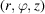a cylindrical polar coordinate system in real space, in which the z axis is parallel to the molecular axes. The molecule is said to have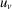helix symmetry, where u and v are integers, if the electron density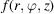satisfies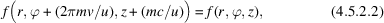where m is any integer. The constant c is the period along the z direction, which is referred to variously as the molecular repeat distance, the crystallographic repeat, or the c repeat. The helix pitch P is equal to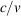. Helix symmetry is easily interpreted as follows. There are u subunits, or helix repeat units, in one c repeat of the molecule. The helix repeat units are repeated by integral rotations of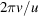about, and translations of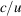along, the molecular (or helix) axis. The helix repeat units may therefore be referenced to a helical lattice that consists of points at a fixed radius, with relative rotations and translations as described above. These points lie on a helix of pitch P, there are v turns (or pitch-lengths) of the helix in one c repeat, and there are u helical lattice points in one c repeat. Ahelix is said to have `u residues in v turns'.

Since the electron density is periodic in ϕ and z, it can be decomposed into a Fourier series as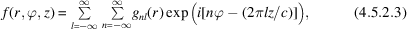where the coefficients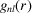are given by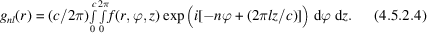Assume now that the electron density has helical symmetry. Denote by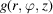the electron density in the region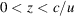; the electron density being zero outside this region, i.e.is the electron density of a single helix repeat unit. It follows that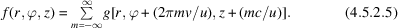Substituting equation (4.5.2.5)into equation (4.5.2.4)shows thatvanishes unless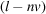is a multiple of u, i.e. unless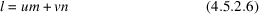for any integer m. Equation (4.5.2.6)is called the helix selection rule. The electron density in the helix repeat unit is therefore given by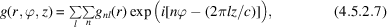where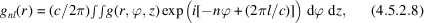and where in equation (4.5.2.7)(and in the remainder of this section) the sum over l is over all integers, the sum over n is over all integers satisfying the helix selection rule and the integral in equation (4.5.2.8)is over one helix repeat unit. The effect of helix symmetry, therefore, is to restrict the number of Fourier coefficientsrequired to represent the electron density to those whose index n satisfies the selection rule. Note that the selection rule is usually derived using a rather more complicated argument by considering the convolution of the Fourier transform of a continuous filamentary helix with a set of planes in reciprocal space (Cochran et al., 1952). The approach described above, which follows that of Millane (1991), is much more straightforward.

### ReferencesCochran, W., Crick, F. H. C. & Vand, V. (1952). The structure of synthetic polypeptides. I. The transform of atoms on a helix. Acta Cryst. 5, 581–586.Google ScholarMillane, R. P. (1991). An alternative approach to helical diffraction. Acta Cryst. A47, 449–451.Google Scholar